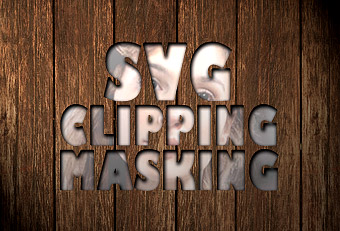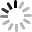# SVG 研究之路 (9) - Clipping and Masking## Cliping

``````<defs>
<clipPath id="a1">
<rect x="0" y="0" width="200" height="100" />
</clipPath>
</defs>
<circle cx="100" cy="100" r="100" clip-path="url(#a1)" fill="#000" />
````````````<clipPath id="a1">
<polygon id="a1Shape" points="100,10 40,180 190,60 10,60 160,180 100,10" stroke="blue" />
</clipPath>

<clipPath id="a2">
<circle id="a2Shape" cx="100" cy="100" r="65" />
</clipPath>

<!-- Intersection -->
<clipPath id="b1" clip-path="url(#a1)">
<use x="0" y="0" width="200" height="200" xlink:href="#a2Shape" />
</clipPath>

<clipPath id="b2">
<use x="0" y="0" width="200" height="200" xlink:href="#a1Shape" />
<use x="0" y="0" width="200" height="200" xlink:href="#a2Shape" />
</clipPath>

<rect x="10" y="10" width="180" height="180" fill="#c00"
clip-path="url(#a1)" />
<rect x="10" y="10" width="180" height="180" fill="#0a0"
clip-path="url(#a2)" transform="translate(200)"/>
<rect x="10" y="10" width="180" height="180" fill="#09c"
clip-path="url(#b1)" transform="translate(400)" />
<rect x="10" y="10" width="180" height="180" fill="#f90"
clip-path="url(#b2)" transform="translate(600)" />
````````````<rect id="box" x="50" y="50" width="50" height="50" fill="#069"/>
<use xlink:href="#box" x="50" y="50" style="fill:#f00;" />
````````````<rect id="box" x="50" y="50" width="50" height="50"/>
<use xlink:href="#box" x="50" y="50" style="fill:#f00;" />
````````````<defs>
<rect  x="50" y="50" width="100" height="100" fill="#ccc"/>
<rect  x="150" y="150" width="50" height="50" fill="#fff"/>
</defs>
<rect id="box1" x="50" y="50" width="150" height="150" fill="#0f0"/>
````````````<defs>
<rect  x="0" y="0" width="50" height="50" fill="#fff"/>
<rect  x="50" y="0" width="50" height="50" fill="#bbb"/>
<rect  x="100" y="0" width="50" height="50" fill="#999"/>
<rect  x="150" y="0" width="50" height="50" fill="#666"/>
<rect  x="200" y="0" width="50" height="50" fill="#222"/>
</defs>
````````````<defs>
<stop offset="0" stop-color="white" stop-opacity="0" />
<stop offset="1" stop-color="white" stop-opacity="1" />